# How to Calculate and Solve for Water Activity | Ball Mill Sizing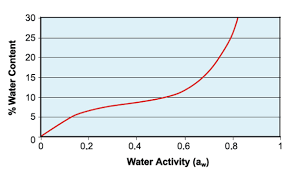The image above represents water activity.

To compute for water activity, two essential parameters are needed and these parameters are Partial Pressure of Water (Pw) and Equilibrium Vapour Pressure of Water at Same Temperature (V.P.w).

The formula for calculating water activity:

aw = Pw/V.P.w

Where:

aw = Water Activity | Drying
Pw = Partial Pressure of Water
V.P.w = Equilibrium Vapour Pressure of Water at Same Temperature

Let’s solve an example;
Find the water activity when the partial pressure of water is 22 and the equilibrium vapour pressure of water at same temperature is 28.

This implies that;

Pw = Partial Pressure of Water = 22
V.P.w = Equilibrium Vapour Pressure of Water at Same Temperature = 28

aw = Pw/V.P.w
aw = 22/28
aw = 0.78

Therefore, the water activity is 0.78.

Calculating the Partial Pressure of Water when the Water Activity and the Equilibrium Vapour Pressure of Water at Same Temperature is Given.

Pw = aw x V.P.w

Where;

Pw = Partial Pressure of Water
aw = Water Activity | Drying
V.P.w = Equilibrium Vapour Pressure of Water at Same Temperature

Let’s solve an example;
Find the partial pressure of water when the water activity is 24 and the equilibrium vapour pressure of water at same temperature is 14.

This implies that;

aw = Water Activity | Drying = 24
V.P.w = Equilibrium Vapour Pressure of Water at Same Temperature = 14

Pw = aw x V.P.w
Pw = 24 x 14
Pw = 336

Therefore, the partial pressure of water is 336.

Calculating the Equilibrium Vapour Pressure of Water at Same Temperature when the Water Activity and the Partial Pressure of Water is Given.

V.P.w = Pw / aw

Where;

V.P.w = Equilibrium Vapour Pressure of Water at Same Temperature
aw = Water Activity | Drying
Pw = Partial Pressure of Water

Let’s solve an example;
Find the equilibrium vapour pressure of water at same temperature when the water activity is 10 and the partial pressure of water is 30.

This implies that;

aw = Water Activity | Drying = 10
Pw = Partial Pressure of Water = 30

V.P.w = Pw / aw
V.P.w = 30 / 10
V.P.w = 3

Therefore, the equilibrium vapour pressure of water at same temperature is 3.

Nickzom Calculator – The Calculator Encyclopedia is capable of calculating the water activity.

To get the answer and workings of the water activity using the Nickzom Calculator – The Calculator Encyclopedia. First, you need to obtain the app.

You can get this app via any of these means:

To get access to the professional version via web, you need to register and subscribe for NGN 2,000 per annum to have utter access to all functionalities.
You can also try the demo version via https://www.nickzom.org/calculator

Apple (Paid) – https://itunes.apple.com/us/app/nickzom-calculator/id1331162702?mt=8
Once, you have obtained the calculator encyclopedia app, proceed to the Calculator Map, then click on Materials and Metallurgical under Engineering.Now, Click on Ball Mill Sizing under Materials and Metallurgical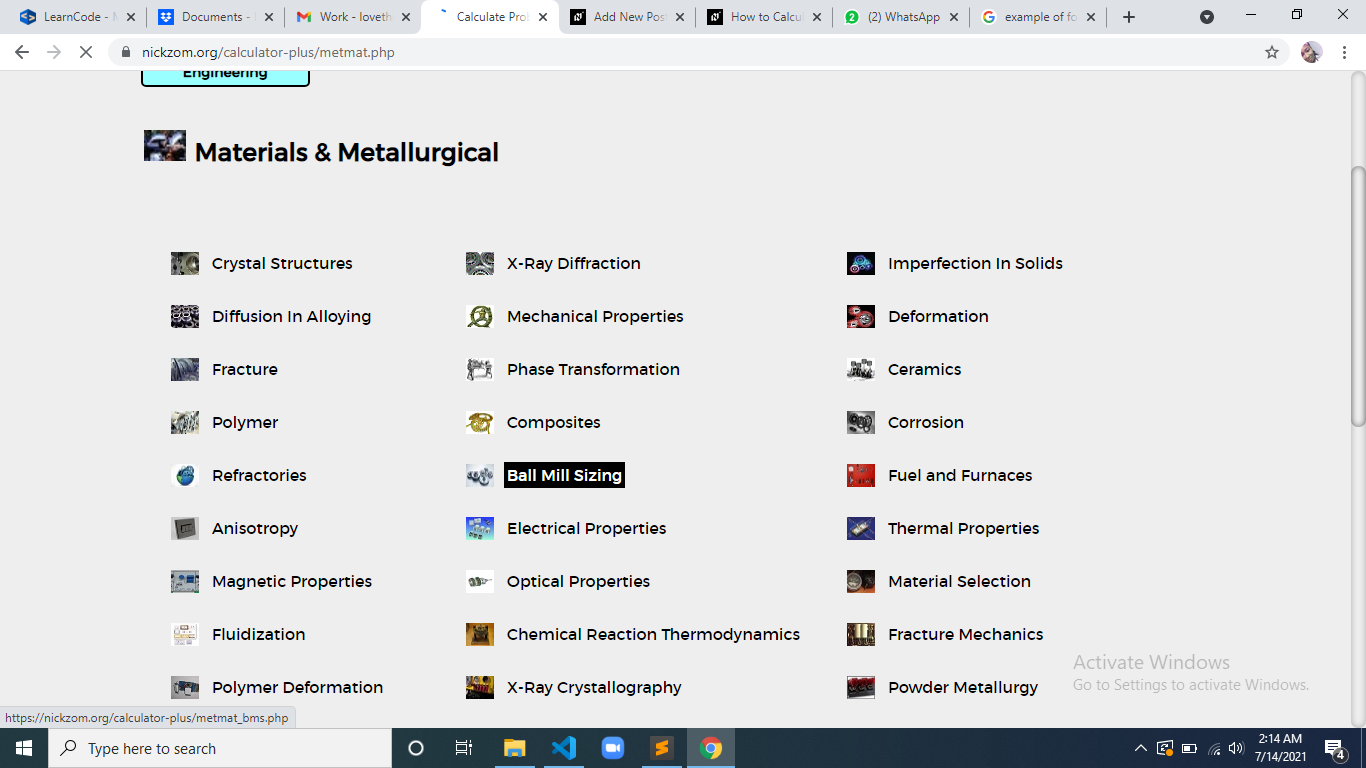Now, Click on Water Activity under Ball Mill Sizing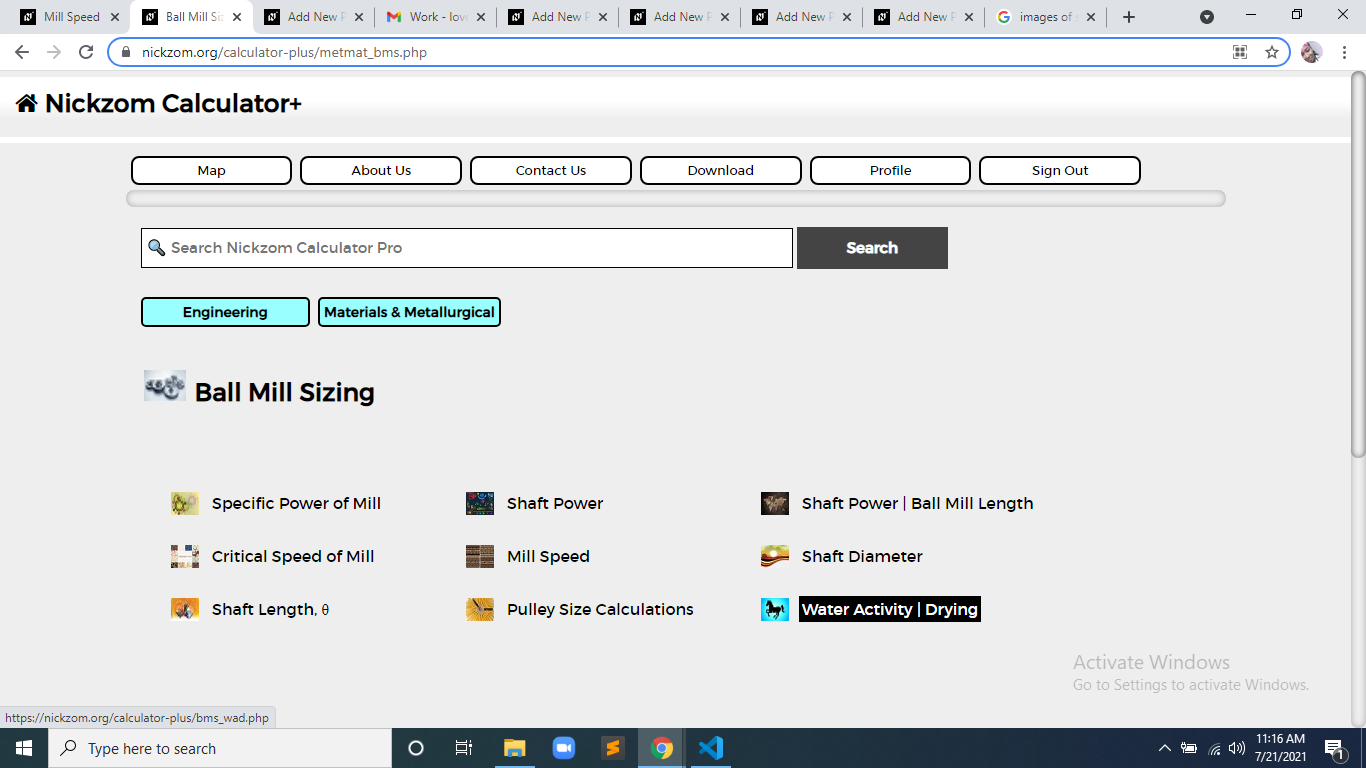The screenshot below displays the page or activity to enter your values, to get the answer for the water activity according to the respective parameters which is the Partial Pressure of Water (Pw) and Equilibrium Vapour Pressure of Water at Same Temperature (V.P.w).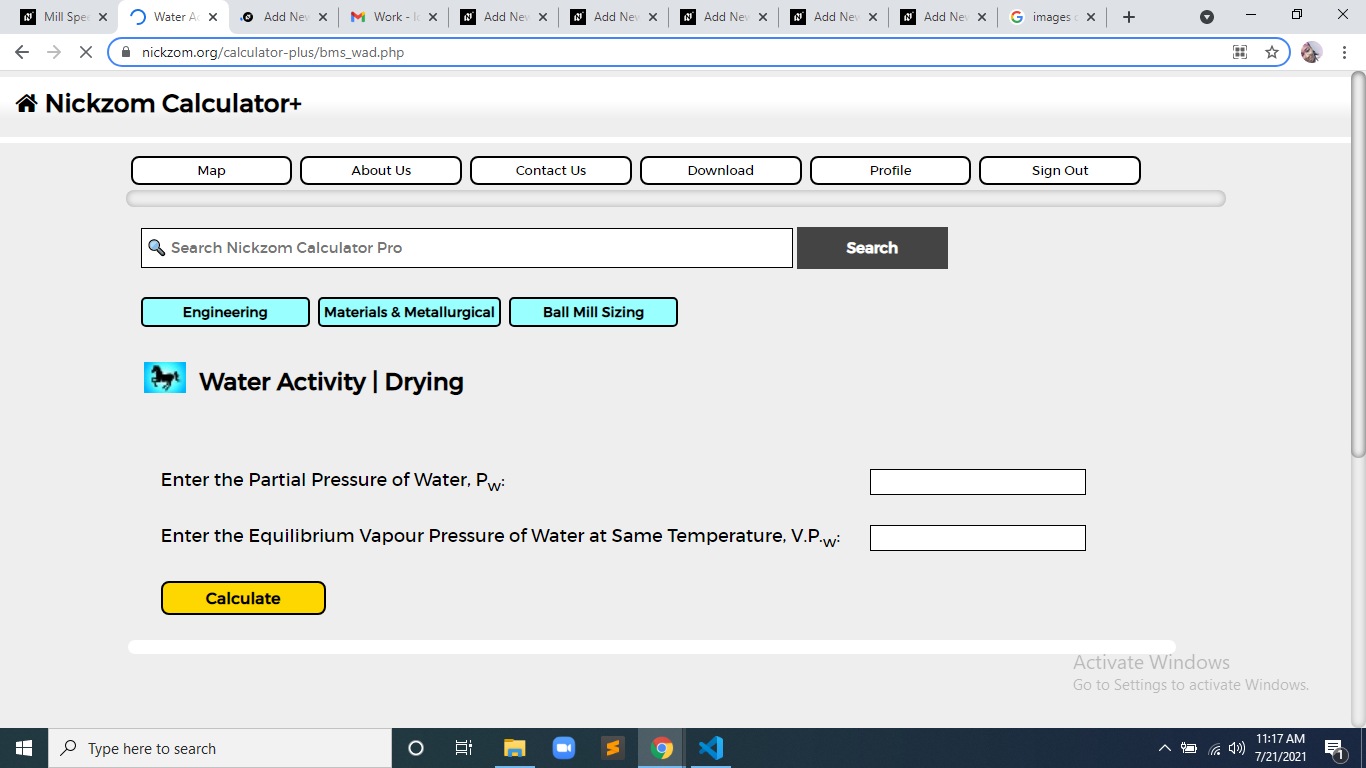Now, enter the value appropriately and accordingly for the parameter as required by the Partial Pressure of Water (Pwis 22 and Equilibrium Vapour Pressure of Water at Same Temperature (V.P.w) is 28.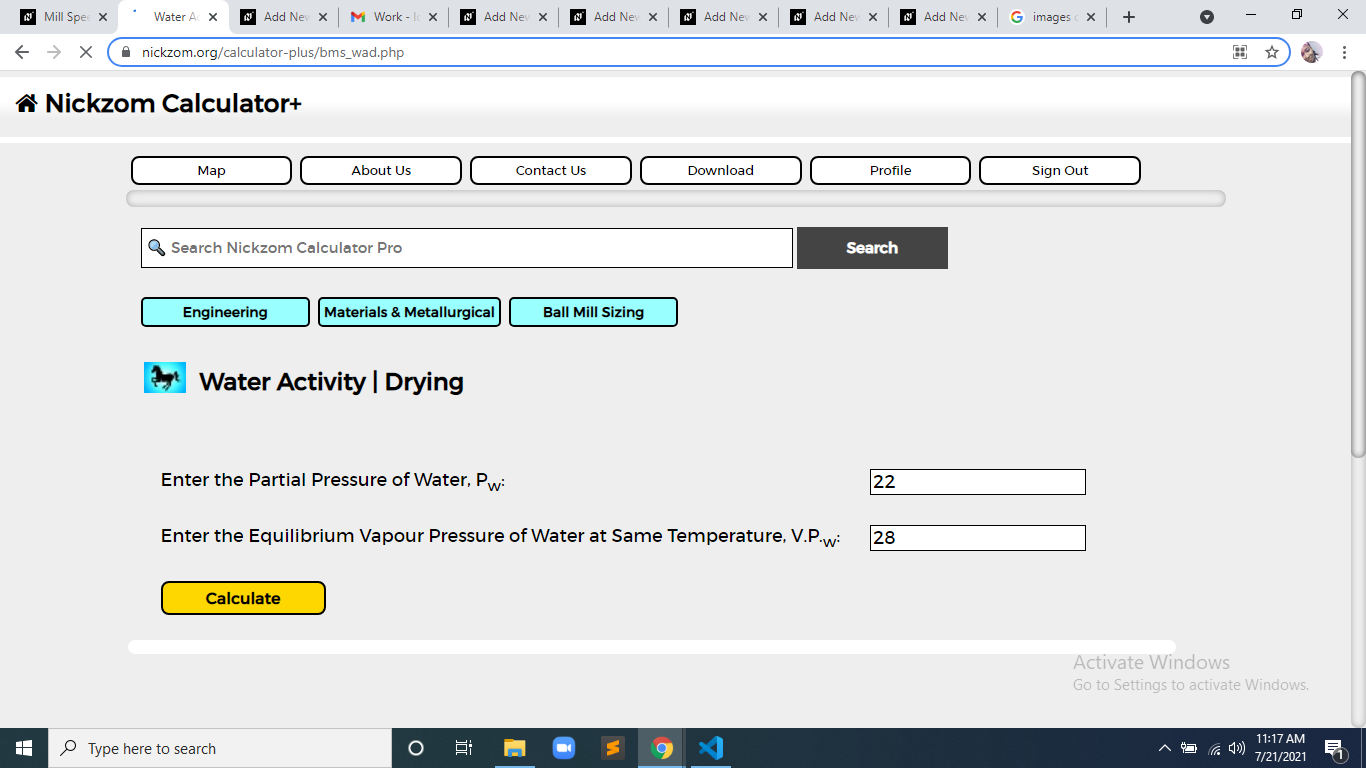Finally, Click on Calculate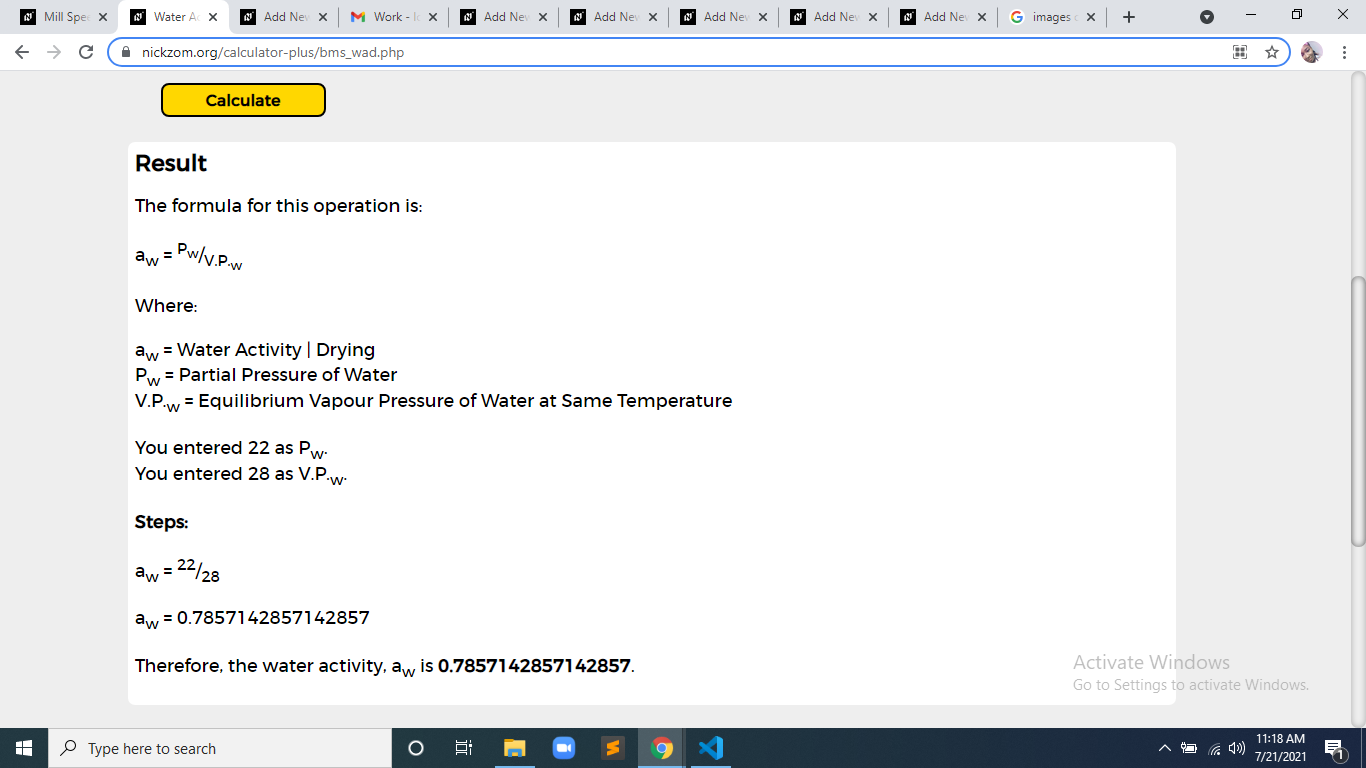As you can see from the screenshot above, Nickzom Calculator– The Calculator Encyclopedia solves for the water activity and presents the formula, workings and steps too.